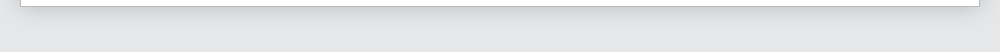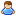Login Products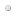SalesSupportDownloadsAbout
 Home » Technical Support » DBISAM Technical Support » Product Manuals » DBISAM Version 4 Manual for RAD Studio XE (Delphi) » SQL Reference » Operators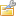Operators

Introduction
DBISAM allows comparison operators, extended comparison operators, arithmetic operators, string operators, date, time, and timestamp operators, and logical operators in SQL statements. These operators are detailed below.

Comparison Operators
Use comparison operators to perform comparisons on data in SELECT, INSERT, UPDATE, or DELETE queries. DBISAM's SQL supports the following comparison operators:

 Operator Description
 < Determines if a value is less than another value. > Determines if a value is greater than another value. = Determines if a value is equal to another value. <> Determines if a value is not equal to another value. >= Determines if a value is greater than or equal to another value. <= Determines if a value is less than or equal to another value.

Use comparison operators to compare two like values. Values compared can be: column values, literals, or calculations. The result of the comparison is a boolean value that is used in contexts like a WHERE clause to determine on a row-by-row basis whether a row meets the filtering criteria. The following example uses the >= comparison operator to show only the orders where the ItemsTotal column is greater than or equal to 1000:

```SELECT *
FROM Orders
WHERE (ItemsTotal >= 1000)```

Comparisons must be between two values of the same or a compatible data type. The result of a comparison operation can be modified by a logical operator, such as NOT. The following example uses the >= comparison operator and the logical NOT operator to show only the orders where the ItemsTotal column is not greater than or equal to 1000:

```SELECT *
FROM Orders
WHERE NOT (ItemsTotal >= 1000)```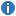Comparison operators can only be used in a WHERE or HAVING clause, or in the ON clause of a join - they cannot be used in the SELECT clause. The only exception to this would be within the first argument to the IF() function, which allows comparison expressions for performing IF...ELSE boolean logic.

Extended Comparison Operators
Use extended comparison operators to perform comparisons on data in SELECT, INSERT, UPDATE, or DELETE queries. DBISAM supports the following extended comparison operators:

 Operator Description
 [NOT] BETWEEN Compares a value to a range formed by two values. [NOT] IN Determines whether a value exists in a list of values. [NOT] LIKE Compares, in part or in whole, one value with another. IS [NOT] NULL Compares a value with an empty, or NULL, value.

BETWEEN Extended Comparison Operator
The BETWEEN extended comparison operator determines whether a value falls inside a range. The syntax is as follows:

`value1 [NOT] BETWEEN value2 AND value3`

Use the BETWEEN extended comparison operator to compare a value to a value range. If the value is greater than or equal to the low end of the range and less than or equal to the high end of the range, BETWEEN returns a TRUE value. If the value is less than the low end value or greater than the high end value, BETWEEN returns a FALSE value. For example, the expression below returns a FALSE value because 10 is not between 1 and 5:

`10 BETWEEN 1 AND 5`

Use NOT to return the converse of a BETWEEN comparison. For example, the expression below returns a TRUE value:

`10 NOT BETWEEN 1 AND 5`

BETWEEN can be used with all non-BLOB data types, but all values compared must be of the same or a compatible data type. The left-side and right-side values used in a BETWEEN comparison may be columns, literals, or calculated values. The following example returns all orders where the SaleDate column is between January 1, 1998 and December 31, 1998:

```SELECT SaleDate
FROM Orders
WHERE (SaleDate BETWEEN '1998-01-01' AND '1998-12-31')```

BETWEEN is useful when filtering to retrieve rows with contiguous values that fall within the specified range. For filtering to retrieve rows with noncontiguous values, use the IN extended comparison operator.

IN Extended Comparison Operator
The IN extended comparison operator indicates whether a value exists in a set of values. The syntax is as follows:

`value [NOT] IN (value_set)`

Use the IN extended comparison operator to filter a table based on the existence of a column value in a specified set of comparison values. The set of comparison values can be a comma-separated list of column names, literals, or calculated values. The following example returns all customers where the State column is either 'CA' or 'HI':

```SELECT c.Company, c.State
FROM Customer c
WHERE (c.State IN ('CA', 'HI'))```

The value to compare with the values set can be any or a combination of a column value, a literal value, or a calculated value. Use NOT to return the converse of an IN comparison. IN can be used with all non-BLOB data types, but all values compared must be of the same or a compatible data type.

IN is useful when filtering to retrieve rows with noncontiguous values. For filtering to retrieve rows with contiguous values that fall within a specified range, use the BETWEEN extended comparison operator.

LIKE Extended Comparison Operator
The LIKE extended comparison operator indicates the similarity of one value as compared to another. The syntax is as follows:

```value [NOT] LIKE [substitution_char] comparison_value
[substitution_char] ESCAPE escape_char```

Use the LIKE extended comparison operator to filter a table based on the similarity of a column value to a comparison value. Use of substitution characters allows the comparison to be based on the whole column value or just a portion. The following example returns all customers where the Company column is equal to 'Adventure Undersea':

```SELECT *
FROM Customer

The wildcard substitution character (%) may be used in the comparison to represent an unknown number of characters. LIKE returns a TRUE when the portion of the column value matches that portion of the comparison value not corresponding to the position of the wildcard character. The wildcard character can appear at the beginning, middle, or end of the comparison value (or multiple combinations of these positions). The following example retrieves rows where the column value begins with 'A' and is followed by any number of any characters. Matching values could include 'Action Club' and 'Adventure Undersea', but not 'Blue Sports':

```SELECT *
FROM Customer
WHERE (Company LIKE 'A%')```

The single-character substitution character (_) may be used in the comparison to represent a single character. LIKE returns a TRUE when the portion of the column value matches that portion of the comparison value not corresponding to the position of the single-character substitution character. The single-character substitution character can appear at the beginning, middle, or end of the comparison value (or multiple combinations of these positions). Use one single-character substitution character for each character to be wild in the filter pattern. The following example retrieves rows where the column value begins with 'b' ends with 'n', with one character of any value between. Matching values could include 'bin' and 'ban', but not 'barn':

```SELECT Words
FROM Dictionary
WHERE (Words LIKE 'b_n')```

The ESCAPE keyword can be used after the comparison to represent an escape character in the comparison value. When an escape character is found in the comparison value, DBISAM will treat the next character after the escape character as a literal and not a wildcard character. This allows for the use of the special wildcard characters as literal search characters in the comparison value. For example, the following example retrieves rows where the column value contains the string constant '10%':

```SELECT ID, Description
FROM Items
WHERE (Description LIKE '%10\%%') ESCAPE '\'```

Use NOT to return the converse of a LIKE comparison. LIKE can be used only with string or compatible data types such as memo columns. The comparison performed by the LIKE extended comparison operator is always case-sensitive.

IS NULL Extended Comparison Operator
The IS NULL extended comparison operator indicates whether a column contains a NULL value. The syntax is as follows:

`column_reference IS [NOT] NULL`

Use the IS NULL extended comparison operator to filter a table based on the specified column containing a NULL (empty) value. The following example returns all customers where the InvoiceDate column is null:

```SELECT *
FROM Customer
WHERE (InvoiceDate IS NULL)```

Use NOT to return the converse of a IS NULL comparison.For a numeric column, a zero value is not the same as a NULL value.

Value Operators
Use value operators to return specific values based upon other expressions in SELECT, INSERT, UPDATE, or DELETE queries. DBISAM supports the following value operators:

 Operator Description
 CASE Evaluates a series of boolean expressions and returns the matching result value.

CASE Value Operator
The CASE value operator can be used in with two different syntaxes, one being the normal syntax while the other being a shorthand syntax. The normal syntax is used to evaluate a series of boolean expressions and return the matching result value for the first boolean expression that returns True, and is as follows:

```CASE
WHEN boolean expression THEN value
[WHEN boolean expression THEN value]
[ELSE] value
END```

The following is an example of the normal CASE syntax. It translate a credit card type into a more verbose description:

```SELECT CardType,
CASE
WHEN Upper(CardType)='A' THEN 'American Express'
WHEN Upper(CardType)='M' THEN 'Mastercard'
WHEN Upper(CardType)='V' THEN 'Visa'
WHEN Upper(CardType)='D' THEN 'Diners Club'
END AS CardDesc,
SUM(SalesAmount) AS TotalSales
FROM Transactions
GROUP BY CardType
ORDER BY TotalSales DESC```

The shorthand syntax is as follows:

```CASE expression
WHEN expression THEN value
[WHEN expression THEN value]
[ELSE] value
END```

The primary difference between the shorthand syntax and the normal syntax is the inclusion of the expression directly after the CASE operator itself. It is used as the comparison value for every WHEN expression. All WHEN expressions must be type-compatible with this expression and can be any type, unlike the normal syntax which requires boolean expressions. The rest of the shorthand syntax is the same as the normal syntax.

The following is the above credit card type example using the shorthand syntax:

```SELECT CardType,
CASE Upper(CardType)
WHEN 'A' THEN 'American Express'
WHEN 'M' THEN 'Mastercard'
WHEN 'V' THEN 'Visa'
WHEN 'D' THEN 'Diners Club'
END AS CardDesc,
SUM(SalesAmount) AS TotalSales
FROM Transactions
GROUP BY CardType
ORDER BY TotalSales DESC```

Arithmetic Operators
Use arithmetic operators to perform arithmetic calculations on data in SELECT, INSERT, UPDATE, or DELETE queries. DBISAM's SQL supports the following arithmetic operators:

 Operator Description
 + Add two numeric values together numeric value. - Subtract one numeric value from another numeric value. * Multiply one numeric value by another numeric value. / Divide one numeric value by another numeric value. MOD Returns the modulus of the two integer arguments as an integer

Calculations can be performed wherever non-aggregated data values are allowed, such as in a SELECT or WHERE clause. In following example, a column value is multiplied by a numeric literal:

```SELECT (itemstotal * 0.0825) AS Tax
FROM orders```

Arithmetic calculations are performed in the normal order of precedence: multiplication, division, modulus, addition, and then subtraction. To cause a calculation to be performed out of the normal order of precedence, use parentheses around the operation to be performed first. In the next example, the addition is performed before the multiplication:

```SELECT (n.numbers * (n.multiple + 1)) AS Result
FROM numbertable n```

Arithmetic operators operate only on numeric values.

String Operators
Use string operators to perform string concatenation on character data in SELECT, INSERT, UPDATE, or DELETE queries. DBISAM's SQL supports the following string operators:

 Operator Description
 + Concatenate two string values together. || Concatenate two string values together.

String operations can be performed wherever non-aggregated data values are allowed, such as in a SELECT or WHERE clause. In following example, a column value concatenated with a second column value to provide a new calculated column in the query result set:

```SELECT (LastName + ', ' + FirstName) AS FullName
FROM Employee```

String operators operate only on string values or memo columns.

Date, Time, and Timestamp Operators
Use date, time, and timestamp operators to perform date, time, and timestamp calculations in SELECT, INSERT, UPDATE, or DELETE queries. DBISAM's SQL supports the following date, time, and timestamp operators:

 Operator Description
 + Adding days or milliseconds to date, time, or timestamp values. - Subtracting days or milliseconds from date, time, or timestamp values, or subtracting two date, time, or timestamp values to get the difference in days or millseconds.

The rules for adding or subtracting dates, times, and timestamps in conjunction with integers are as follows:

Adding an integer to a date is equivalent to adding days to the date

Adding an integer to a time is equivalent to adding milliseconds to the time (be careful of wraparound since a time value is equal to the number of milliseconds elapsed since the beginning of the current day)

Adding an integer to a timestamp is equivalent to adding milliseconds to the time portion of the timestamp (any milliseconds beyond the number of milliseconds in a day will result in an increment of the day value in the timestamp by 1)

Subtracting an integer from a date is equivalent to subtracting days from the date

Subtracting an integer from a time is equivalent to subtracting milliseconds from the time (be careful of going below 0, which will be ignored)

Subtracting an integer from a timestamp is equivalent to subtracting milliseconds from the time portion of the timestamp (any milliseconds less than 0 for the time portion will result in a decrement of the day value in the timestamp by 1)

Subtracting a date value from another date value will result in the number of days between the two dates (be sure to use the ABS() function to ensure a positive value if the second value is larger than the first)

Subtracting a time value from another time value will result in the number of milliseconds between the two times (be sure to use the ABS() function to ensure a positive value if the second value is larger than the first)

Subtracting a date value from a timestamp value will result in the number of milliseconds between the timestamp and the date (be sure to use the ABS() function to ensure a positive value if the second value is larger than the first)

Subtracting a timestamp value from a timestamp value will result in the number of milliseconds between the timestamp and the other timestamp (be sure to use the ABS() function to ensure a positive value if the second value is larger than the first)

The following example shows how you would add 30 days to a date to get an invoice due date for an invoice in a SELECT SQL statement:

```SELECT InvoiceDate, (InvoiceDate + 30) AS DueDate, BalanceDue
FROM Invoices
WHERE InvoiceDate BETWEEN '1999-01-01' AND '1999-01-31'```

Date, time, and timestamp operators operate only on date, time, or timestamp values in conjuction with integer values.

Logical Operators
Use logical operators to perform Boolean logic between different predicates (conditions) in an SQL WHERE clause. DBISAM's SQL supports the following logical operators:

 Operator Description
 NOT NOT a boolean value. AND AND two boolean values together. OR OR two boolean values together.

This allows the source table(s) to be filtered based on multiple conditions. Logical operators compare the boolean result of two predicate comparisons, each producing a boolean result. If OR is used, either of the two predicate comparisons can result on a TRUE value for the whole expression to evaluate to TRUE. If AND is used, both predicate comparisons must evaluate to TRUE for the whole expression to be TRUE; if either is FALSE, the whole is FALSE. In the following example, if only one of the two predicate comparisons is TRUE, the row will be included in the query result set:

```SELECT *
FROM Reservations
WHERE ((ReservationDate < '1998-01-31') OR (Paid = TRUE))```

Logical operator comparisons are performed in the order of AND and then OR. To perform a comparison out of the normal order of precedence, use parentheses around the comparison to be performed first. The SELECT statement below retrieves all rows where the Shape column is 'Round' and the Color 'Blue':

```SELECT Shape, Color
FROM Objects
WHERE (Color = 'Red' OR Shape = 'Round') AND Color = 'Blue'```

Without the parentheses, the default order of precedence is used and the logic changes. The next example, a variation on the above statement, would return rows where the Shape is 'Round' and the Color is 'Blue', but would also return rows where the Color is 'Red', regardless of the Shape:

```SELECT Shape, Color
FROM Objects
WHERE Color = 'Red' OR Shape = 'Round' AND Color = 'Blue'```

Use the NOT operator to negate the boolean result of a comparison. In the following example, only those rows where the Paid column contains a FALSE value are retrieved:

```SELECT *
FROM reservations
WHERE (NOT (Paid = TRUE))```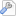More Support Options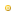Support ForumsFrequently Asked QuestionsTechnical BulletinsTechnical ArticlesIncident ReportsRoadmap